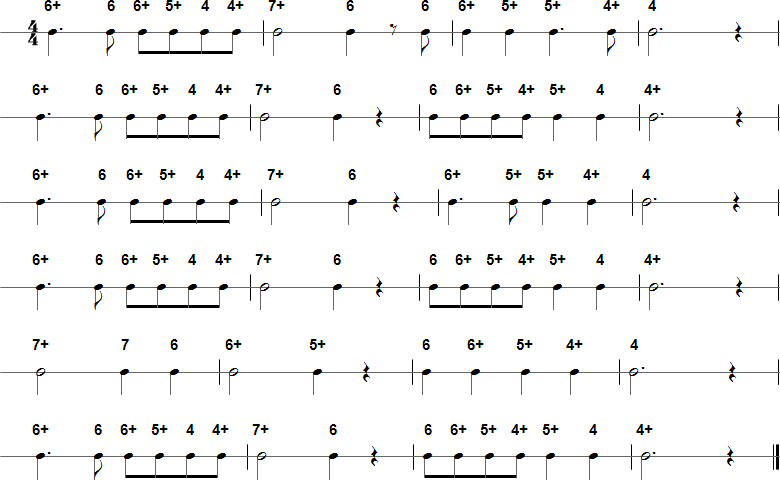Browse by Title:

# Massa's in the Cold Ground - Harmonica Tablature and Sheet Music## Tab in Text Format

6+ 6 6+ 5+ 4 4+ 7+ 6 6 6+ 5+ 5+ 4+ 4 6+ 6 6+ 5+ 4 4+ 7+ 6 6 6+ 5+ 4+ 5+ 4 4+ 6+ 6 6+ 5+ 4 4+ 7+ 6 6+ 5+ 5+ 4+ 4 6+ 6 6+ 5+ 4 4+ 7+ 6 6 6+ 5+ 4+ 5+ 4 4+ 7+ 7 6 6+ 5+ 6 6+ 5+ 4+ 4 6+ 6 6+ 5+ 4 4+ 7+ 6 6 6+ 5+ 4+ 5+ 4 4+